# Errors in MC and MD

Williams and Holland's Law:

If enough data is collected, anything may be proven by statistical methods.

Suppose we are analyzing the set of configurations, produced in either MC or MD simulations. The total number of configurations isThe time average of a property A is then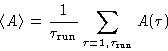(1)
If each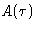were statistically independent of the others, the variance of the mean would simply be: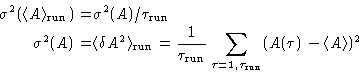(2)
and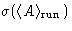gives the estimated error in average. But usually the data produce in MD and MC simulations are correlated. As a limiting case we can assume, that our set ofconfigurations actually consists of blocks of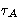identical values (Jacucci and Rahman, 1984,corresponds to the correlation time in the MD simulations). Then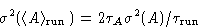(3)
To estimate the real error bars we split our data in blocks, varying the block length, calculate the block averages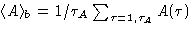and then estimate the variance, using these block averages: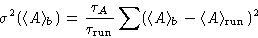(4)
As the block size increases, block averages become less and less correlated, and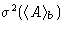becomes proportional to the number of blocks, or inversely proportional to. This means, that the value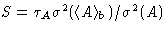becomes independent of. Each S-th configuration of all produced can be taken as a set of uncorrelated configurations. Thus the error estimate should be based on the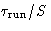configurations, and notitself. That is why efficient MC algorithms are developed to produced less correlated configurations in the same total number of MC steps.Next: Hints for Using Program Up: Appendix: Some Advice for Previous: Appendix: Some Advice for

© 1997 Boris Veytsman and Michael Kotelyanskii
Tue Dec 2 20:15:24 EST 1997